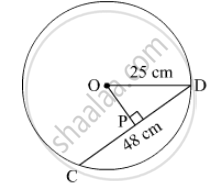# Radius of a Circle with Centre O is 25 Cm. Find the Distance of a Chord from the Centre If Length of the Chord is 48 Cm. - Mathematics

Sum

Radius of a circle with centre O is 25 cm. Find the distance of a chord from the centre if length of the chord is 48 cm.#### Solution

Perpendicular drawn from the centre of the circle to the chord bisects the chord.

So, PD = 1/2 CD = 48/2 = 24 cm

In Δ OPD,

We apply the pythagoras theorem,

OP² + PD² = OD²

⇒ OP² + 24² = 25²

⇒ OP² = 25² - 24² = 625 -576 = 49

⇒ OP² = 49

⇒ OP = 7 CM

Concept: Properties of Chord of a Circle
Is there an error in this question or solution?

#### APPEARS IN

Balbharati Mathematics 8th Standard Maharashtra State Board
Chapter 17 Circle : Chord and Arc
Practice Set 17.1 | Q 2 | Page 116